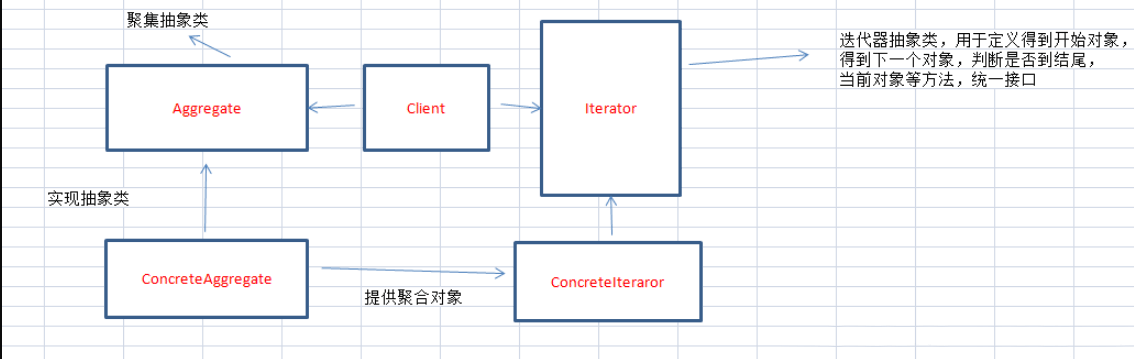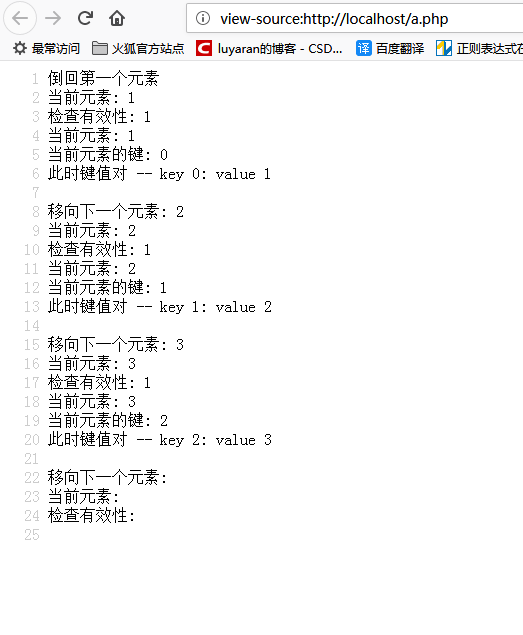# PHP设计模式之迭代器（Iterator）模式入门与应用详解

更新时间：2019年12月09日 10:35:02   作者：luyaran我要评论• Iterator::current ― Return the current element 返回当前元素
• Iterator::key ― Return the key of the current element 返回当前元素的键
• Iterator::next ― Move forward to next element 移向下一个元素
• Iterator::rewind ― Rewind the Iterator to the first element 重新回到第一个元素
• Iterator::valid ― Checks if current position is valid 检查当前位置的有效性

```class MyIterator implements Iterator
{
private \$var = array();

public function __construct(\$array)
{
if (is_array(\$array)) {
\$this->var = \$array;
}
}

public function rewind() {
echo "倒回第一个元素\n";
reset(\$this->var);
}

public function current() {
\$var = current(\$this->var);
echo "当前元素: \$var\n";
return \$var;
}

public function key() {
\$var = key(\$this->var);
echo "当前元素的键: \$var\n";
return \$var;
}

public function next() {
\$var = next(\$this->var);
echo "移向下一个元素: \$var\n";
return \$var;
}

public function valid() {
\$var = \$this->current() !== false;
echo "检查有效性: {\$var}\n";
return \$var;
}
}

\$values = array(1,2,3);
\$it = new MyIterator(\$values);
foreach (\$it as \$k => \$v) {
print "此时键值对 -- key \$k: value \$v\n\n";
}

```1.支持多种遍历方式。比如有序列表，我们根据需要提供正序遍历、倒序遍历两种迭代器。用户只需要得到我们的迭代器，就可以对集合执行遍历操作

2.简化了聚合类。由于引入了迭代器，原有的集合对象不需要自行遍历集合元素了

3.增加新的聚合类和迭代器类很方便，两个维度上可各自独立变化

4.为不同的集合结构提供一个统一的接口，从而支持同样的算法在不同的集合结构上操作

1.访问一个聚合对象内容而无须暴露它的内部显示

2.需要为聚合对象提供多种遍历方式

3.为遍历不同的聚合结构提供一个统一的接口

```Iterator extends Traversable {
/* 方法 */
abstract public mixed current ( void )//返回当前元素
abstract public scalar key ( void )//返回当前元素的键
abstract public void next ( void )//向前移动到下一个元素
abstract public void rewind ( void )//返回到迭代器的第一个元素
abstract public boolean valid ( void )//检查当前位置是否有效
}

```

```class Season implements Iterator{
private \$position = 0;//指针指向0
private \$arr = array('春','夏','秋','冬');
public function rewind(){
return \$this -> position = 0;
}
public function current(){
return \$this -> arr[\$this -> position];
}
public function key(){
return \$this -> position;
}
public function next() {
++\$this -> position;
}

public function valid() {
return isset(\$this -> arr[\$this -> position]);
}
}
\$obj = new Season;
foreach (\$obj as \$key => \$value) {
echo \$key.':'.\$value."\n";
}

```

```class Fibonacci implements Iterator {
private \$previous = 1;
private \$current = 0;
private \$key = 0;

public function current() {
return \$this->current;
}

public function key() {
return \$this->key;
}

public function next() {
// 关键在这里
// 将当前值保存到 \$newprevious
\$newprevious = \$this->current;
// 将上一个值与当前值的和赋给当前值
\$this->current += \$this->previous;
// 前一个当前值赋给上一个值
\$this->previous = \$newprevious;
\$this->key++;
}

public function rewind() {
\$this->previous = 1;
\$this->current = 0;
\$this->key = 0;
}

public function valid() {
return true;
}
}

\$seq = new Fibonacci;
\$i = 0;
foreach (\$seq as \$f) {
echo "\$f ";
if (\$i++ === 15) break;
}

```

0 1 1 2 3 5 8 13 21 34 55 89 144 233 377 610